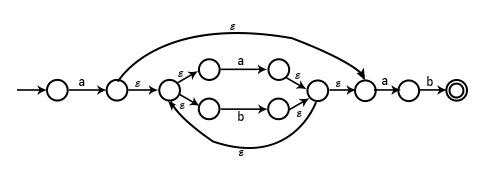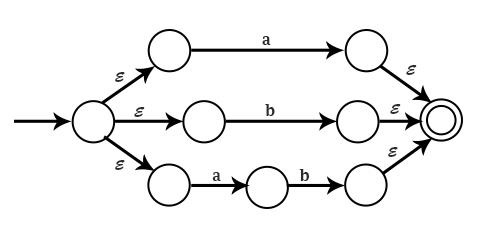# What is Non-Deterministic Finite Automata (NFA)?

Non-Deterministic means that there can be several possible transitions on the same input symbol from some state. The output is non-deterministic for a given input. NFA can be represented as a nondeterministic finite state machine.

NFA can be represented by 5 tuples (Q,$\sum$,$\delta$,q0,F)

• Q is a finite non-empty set of states.

• $\sum$ is a finite set of input symbols.

• $\delta$ is the transition function to move from the current state to the next state.

∴ $\delta$:Q x $\sum$ → 2Q

• q0 ∈ Q is the initial state.

• F \subseteq Q is the set of final states.

Example1 − Draw NFA for the Regular Expression a(a+b)*ab

SolutionExample2 − Draw NFA for a + b + ab

SolutionExample3 − Let M=(Q,∑,$\delta$,q0,F) be an NFA. Show that for any qϵQ and a ϵ Σ,$\delta$′(q,a)= $\delta$(q,a)

Solution

Let M=(Q,∑,$\delta$,q0,F) be NFA that recognizes the language L. Then the DFA, M′=(Q1,∑,$\delta$′,q′0,F′) that satisfies the following conditions recognizes L:Q1=2Q, that is the set of all subset of Q.

q′0={q0}

$\delta$(q,a)=∪pϵq$\delta$(p,a) for each state q in Q1 and each symbol a in Σand

F={qϵQ1|q∩F2≠ф}

To obtain DFA M′=(Q1,Σ,$\delta$′,q′0,F′) which accepts the same language, as given NFA, M=(Q,∑,$\delta$,q0,F) does. It can proceed as follows −

Step1 − Initially Q1

Step2 − Put {q0} into Q1.{q0} is the initial state of the DFA M′.

Step3 − Then for each state q in Q1 do the following: add this new state, add δ(q,a)=∪pϵqδ(p,a) to δ, where δ on the right-hand side is that of NFA M’.

Step4 − Repeat step3 till new states are there to add in Q1, if there is a new state create to add in Q1, the process eliminates.

Example4 − Prove that if L is accepted by an NFA with ∈−transitions, then L is accepted by an NFA without ∈−transitions.

Solution

Suppose L = L (D) for some DFA or NFA without ∈−transitions. It can turn D into a ∈−DFA (E) by adding transitions δ(q,∈)=ф for all states q of D. It can also move the transitions of D on input symbols, for example, δD(q,a)=D into an NFA transition to the set including the only P that is δE(q,a)={P}. Therefore, the transitions of E and D are equal, but E explicitly states that there are no transitions out of any state of E.

Let E=(QE,Σ,δE,q0,FE) be a ∈−NFA. It can use the modified subset construction represented above to make the DFA.

D=(QE,∑,δE,qD,FD)

L(D)=L(E), because the extended transition functions of E and D are equal. The extended transition function is the function that takes a state q and a string w and returns a state P, the state that automation reaches when starting in state q and processing the sequence of inputs w.

δE(q0,w)=δD(qD,w)by induction on the length of w.